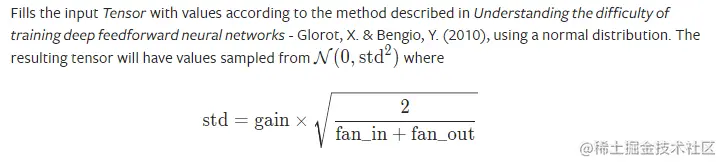## 1.1 均匀分布初始化（uniform_）

torch.nn.init.uniform_(tensor, a=0.0, b=1.0)复制代码
• tensor——一个n维的torch.Tensor
• a – 均匀分布的下界
• b – 均匀分布的上限

## 1.2 正态分布初始化（normal_）

torch.nn.init.normal_(tensor, mean=0.0, std=1.0)复制代码
• tensor——一个n维的torch.Tensor
• mean – 正态分布的均值
• std – 正态分布的标准偏差

## 1.3 常数初始化（constant_）

torch.nn.init.constant_(tensor, val)复制代码
• tensor——一个n维的torch.Tensor
• val – 用来填充张量的值

## 1.4 一值初始化（ones_）

torch.nn.init.ones_(tensor)复制代码

## 1.5 零值初始化（zeros_）

torch.nn.init.zeros_(tensor)复制代码

## 1.6 单位矩阵初始化（eye_）

torch.nn.init.eye_(tensor)复制代码

## 1.7 狄拉克初始化（dirac_）

torch.nn.init.dirac_(tensor, groups=1)复制代码
• tensor – {3, 4, 5} 维torch.Tensor
• groups (optional) – conv 层中的组数（默认值：1）

## 1.8 正交初始化（orthogonal_）

torch.nn.init.orthogonal_(tensor, gain=1)复制代码

## 1.9 稀疏初始化（sparse_）

torch.nn.init.sparse_(tensor, sparsity, std=0.01)复制代码
• tensor——一个n维的torch.Tensor
• sparsity - 每列中要设置为零的元素的比例
• std – 用于生成非零值的正态分布的标准偏差

## 1.10 Xavier初始化

Xavier 初始化方法，论文在《Understanding the difficulty of training deep feedforward neural networks》。公式推导是从“方差一致性”出发，初始化的分布有均匀分布和正态分布两种。

### 1.10.1 Xavier均匀分布（xavier_uniform_）

torch.nn.init.xavier_uniform_(tensor, gain=1.0)复制代码

xavier 初始化方法中服从均匀分布 U(−a,a) ，分布的参数 a = gain * sqrt(6/fan_in+fan_out)，eg：

nn.init.xavier_uniform_(w, gain=nn.init.calculate_gain('relu'))复制代码

### 1.10.2 Xavier正态分布（xavier_normal_）

torch.nn.init.xavier_normal_(tensor, gain=1.0)复制代码

xavier 初始化方法中服从正态分布，mean=0,std = gain * sqrt(2/fan_in + fan_out)## 1.11 kaiming初始化

kaiming 初始化方法，论文在《 Delving deep into rectifiers: Surpassing human-level performance on ImageNet classification 》，公式推导同样从“方差一致性”出法，kaiming是针对 xavier 初始化方法在 relu 这一类激活函数表现不佳而提出的改进

### 1.11.1 kaiming均匀分布（kaiming_uniform_）

torch.nn.init.kaiming_uniform_(tensor, a=0, mode='fan_in', nonlinearity='leaky_relu')复制代码
• tensor——一个n维的torch.Tensor
• a – 为激活函数的负半轴的斜率（仅与“leaky_relu”一起使用）,relu 是 0
• mode——“fan_in”（默认）或“fan_out”。 选择“fan_in”会保留前向传递中权重方差的大小。 选择“fan_out”会保留向后传递的幅度。
• nonlinearity – 非线性函数（nn.functional 名称），建议仅与 'relu' 或 'leaky_relu'（默认）一起使用。### 1.11.2 kaiming正态分布（kaiming_normal_）

torch.nn.init.kaiming_normal_(tensor, a=0, mode='fan_in', nonlinearity='leaky_relu')复制代码
• tensor——一个n维的torch.Tensor
• a – 为激活函数的负半轴的斜率（仅与“leaky_relu”一起使用）,relu 是 0
• mode——可选为 fan_in 或 fan_out, fan_in 使正向传播时，方差一致;fan_out 使反向传播时，方差一致
• nonlinearity – 非线性函数（nn.functional 名称），建议仅与 'relu' 或 'leaky_relu'（默认）一起使用。eg：

nn.init.kaiming_normal_(w, mode='fan_out', nonlinearity='relu')复制代码

## 1.12 计算增益

torch.nn.init.calculate_gain(nonlinearity, param=None)复制代码
• nonlinearity–非线性函数(nn.functional name)
• param–非线性函数的可选参数